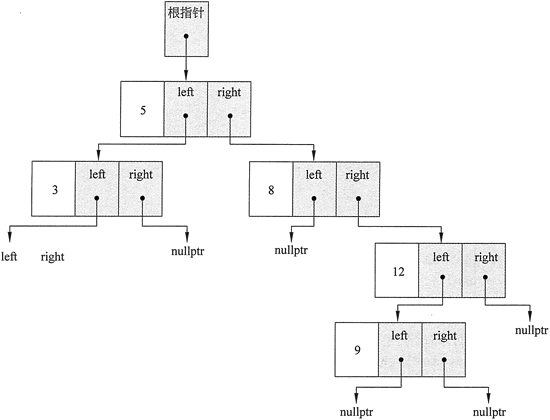• 内容
• 评论
• 相关

## 创建二叉搜索树

```class TreeNode
{
int value;
TreeNode *left;
TreeNode *right;
TreeNode(int value1,TreeNode *left1 = nullptr,TreeNode *right1 = nullptr)
{
value = value1;
left = left1;
right = right1;
}
};```

```//IntBinaryTree.h的内容
class IntBinaryTree
{
private:
//The TreeNode struct is used to build the tree.
struct TreeNode
{
int value;
TreeNode *left;
TreeNode *right;
TreeNode(int value1,TreeNode *left1 = nullptr,TreeNode *right1 = nullptr)
{
value = value1;
left = left1;
right = right1;
}
};
TreeNode *root; // Pointer to the root of the tree
// Various helper member functions.
void insert(TreeNode *&, int);
void destroySubtree(TreeNode *);
void remove(TreeNode *&, int);
void makeDeletion(TreeNode *&);
void displayInOrder(TreeNode *) const;
void displayPreOrder(TreeNode *) const;
void displayPostOrder(TreeNode *) const;
public:
// These member functions are the public interface.
IntBinaryTree。    // Constructor
{
root = nullptr;
}
~IntBinaryTree()    // Destructor
{
destroySubtree(root);
}
void insert(int num)
{
insert (root, num);
}
bool search(int) const;
void remove(int num)
{
remove(root, num);
}
void showInOrder(void) const
{
displayInOrder(root);
}
void showPreOrder() const
{
displayPreOrder(root);
}
void showPostOrder() const
{
displayPostOrder(root);
}
}```

TreeNode *tree;

TreeNode *root;

IntBinaryTree Tree;

IntBinaryTree 的公共成员函数包括：构造函数、析构函数、用于在树中插入新数字的成员函数、用于搜索树以确定给定的数字是否在树中的成员函数、用于从树中移除数字的成员函数，以及根据不同的顺序显示存储在树中的数字的成员函数。

```// This program builds a binary tree with 5 nodes.
#include <iostream>
#include "IntBinaryTree.h"
using namespace std;

int main()
{
IntBinaryTree tree;
cout << "Inserting numbers. ";
tree.insert (5);
tree.insert(8);
tree.insert (3);
tree.insert(12);
tree.insert (9);
cout << "Done. \n";
return 0;
}```0条评论